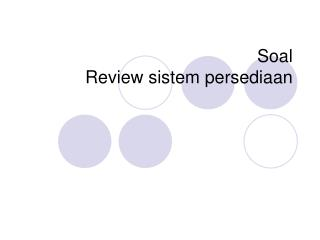DownloadDownload PresentationSoal Review sistem persediaan

# Soal Review sistem persediaan

Télécharger la présentation## Soal Review sistem persediaan

- - - - - - - - - - - - - - - - - - - - - - - - - - - E N D - - - - - - - - - - - - - - - - - - - - - - - - - - -
##### Presentation Transcript

1. Soal Review sistem persediaan

2. Calculate EOQ 2DS 2(Annual Demand)(Order or set-up cost) Qeoq = = H Annual Holding Cost when to place an order. Reorder point R=DL D = Avg daily demand (constant) L = Lead time (constant) • Exercise EOQ and reorder point? • Annual demand = 2,000 units • Days/year in average daily demand = 365 • Cost to place an order = £10 • Holding cost /unit p.a. = £2.50 • Lead time = 7 days • Cost per unit = £15

3. EOQ Solution 2DS 2(1,000 )(10) Q = = = 89.443 units or 90 units eoq H 2.50 1,000 units p.a. d = = 2.74 units/day 365 days p.a. Reorder point D L = 2.74 units/day = 19.18 or 20 for 7 day lead time EOQ order = 90 units. When only 20 units left, place next order for 90 units.

4. 10,000 units/year D = = 27.397 units/day 365 days EOQ and ROQ example 2 Annual Demand = 10,000 units Days per year considered in average daily demand = 365 Cost to place an order = £10 Holding cost per unit per year = 10% of cost per unit Lead time = 10 days Cost per unit = £15 2DS 2(10,000)(10) Q = = = 365.148 (366 units) H 1.50 eoq If lead time = 10 days, ROL= 273.97 = 274 units Place order for 366 units. When 274 left, place next order for 366.

5. Demand 2 x unit cost x Hc% + Oc 1200 2 x £3 x 25% = £450 + £10 Once per year = £460 1200/52 2 x £3 x 25% = £9 + £510 Once per week = £519 approx Total variable cost Avg.stock Find point of minimum TVc

6. EOQ Table – minimum TVc Avg.stock x item £ x hc % Oc + Hc

7. Minimum point of Total Inventory Costs • EOQ = • minimum TVc point Total variable costs £ Costs Total Hc Total Oc EOQ* Order Size (Q)

8. £6.40 x 18.75% EOQ Example Cheapo Bags wants to calculate the EOQ for tapestry cloth used to produce hand bags. • Last year demand = 10,000 metres (constant rate). • Value per metre of tapestry = £6.40 • Oc – each order = £250. • Hc = £1.20 per metre = 18.75% What is the EOQ? 2 x 10,000 x £250 = 2042 metres

9. 2(Demand p.a.)(Order or Setup-cost) QOPT = = 2DS Holding cost per annum iC Price-Break Model Assumptions similar to as EOQ model i = % of unit cost as carrying cost C = cost per unit “C” varies for each price-break so apply the formula to each price-break cost value.

10. 2DS iC Price-Break Example • Brunel University can reduce ordering costs for photocopy paper by placing larger quantity orders. What is the optimal order quantity? • e-mail order cost = £4 • carrying cost % = 2% • Demand p.a. = 10,000 units? Quantity price breaks

11. Solution Put data into formula for each price-break of “C”. D = 10,000 units Order cost (S) = £4 Carrying cost % (i) = 2% Cost per unit (C) = £1.20, £1.00, £0.98 Are Qopt values feasible for the price breaks? 2(10,000)(4) 2(10,000)(4) 2DS = =2,000 = = 1,826 units 0.02(1.00) iC 0.02(1.20) 2(10,000)( 4) = =2,020 0.02(0.98) Qopt 0 - 2499 Feasible 2500-3999 and 4000+ Not feasible

12. U-shaped function True Qopt values occur at the start of each price-break interval.The total annual cost function is a “u” shaped function Total annual costs Price-breaks 0 1826 2500 4000 Order Quantity

13. Price-Break Solution Now apply the Qopt values to total annual cost & identify the total cost for each price-break. TC(0-2499)= (10000x1.20)+(10000/1826)x4+(1826/2)(0.02x1.20) = £12,043.82 TC(2500 -3999) = £10,041 TC(4000+) = £9,949.20 Least cost Qopt = 4000